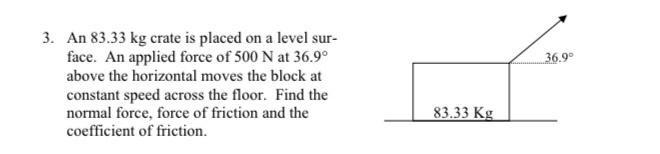Question:

# An 83.33 kg crate is placed on a level sur- face. An applied force of 500 N at 36.9° above the horizontal moves the block at constant speed across the floor. Find the normal force, force of friction aAn 83.33 kg crate is placed on a level sur- face. An applied force of 500 N at 36.9° above the horizontal moves the block at constant speed across the floor. Find the normal force, force of friction and the coefficient of friction.